# 1.引言

• 作者提出了一种简单但是高效的方法来减少DenseNet的冗余性，即将原始bottleneck模块的堆叠数量替换为作者设计的SMG模块，其中SMG模块嵌入了局部的residual连接。
• 此外，SMG模块配备了一个高效的two-stage pipeline，来适应DenseNet-like的结构（需要聚合先前的输出），即：压缩进入的informative但是冗余的feature map通过逐层的卷积，之后激活feature map通过multi-kernel卷积，SMG模块输出的特征是compact并且是具有multi-scale features的。
• 作者进一步通过引入attention机制开发了forget gate和update gate来实现新特征和旧特征的fusion，而不是采用ResNet中简单相加的方式。
• 由于Hybrid Connectivity（嵌套的结合of global dense和local residual，以及Gated mechanism，我们称最终的网络为HCGNet。
• 在CIFAR和Imagenet的实验结果标明HCGNet显著的超过了其他SOTA网络。此外，HCGNet获得了优秀的可解释性和对抗鲁棒性。在MS-COCO数据集上，HCGNet作为backbone，在目标检测和语义分割上超过了ResNet。

# 2.网络结构

## 2.1 Hybrid connectivity混合连通性

### Revisiting ResNet和DenseNet

（1）参数共享

• residual connectivity隐式地伴随着参数共享对于reused features和newly extracted features，因为当前卷积block处理的特征是上一个block的reused features和newly extracted features相加之后的混合特征。
• dense connectivity对于reused features和newly extracted features不是参数共享，因为当前卷积block处理的特征是上一个block的reused features和newly extracted features concate之后的混合特征。

（2）特征学习

• dense connectivity：后来提出的DenseNet开发了全局的dense connectivity，可以将早期的feature maps传送到后续的模块，因此保护了先前的所有信息并且促进了现有feature 的开发。此外，不同的模块对相同的特征进行共同学习，促进了有效的特征探索。

（3）整体效率

### Hybrid connectivity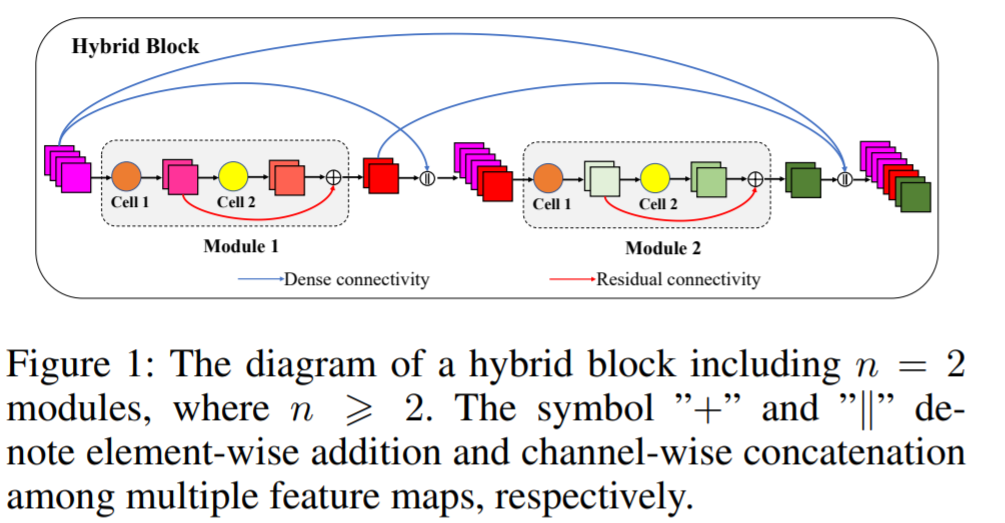hybrid connectivity模式存在于hybrid block中，类似于DenseNet的dense connectivity发生在dense block中。hybrid block包含了 n ( n ≥ 2 ) n(n\geq 2) 个模块，在这里，模块被命名为SMG模块。匹配growth rate在DenseNet的概念，每一个模块产生 k k 个channel的feature map。SMG模块包含2个连续的cell，称为cell 1和cell 2。全局地，每一个模块的输入时先前所有模块的输出的concatenation。局部地，residual connectivity提供了一个shortcut来让cell 1的输出绕过cell 2然后与cell 2产生的新特征进行相加来产生输出。

## 2.2 SMG模块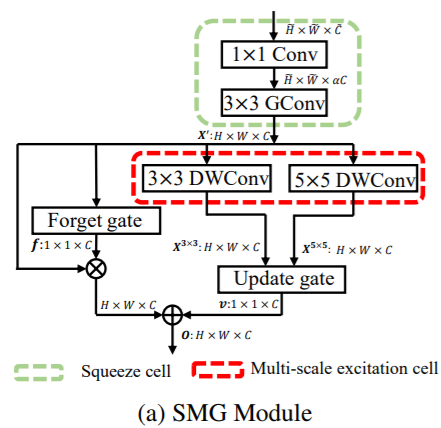### Gate机制

#### Update Gate （更新门）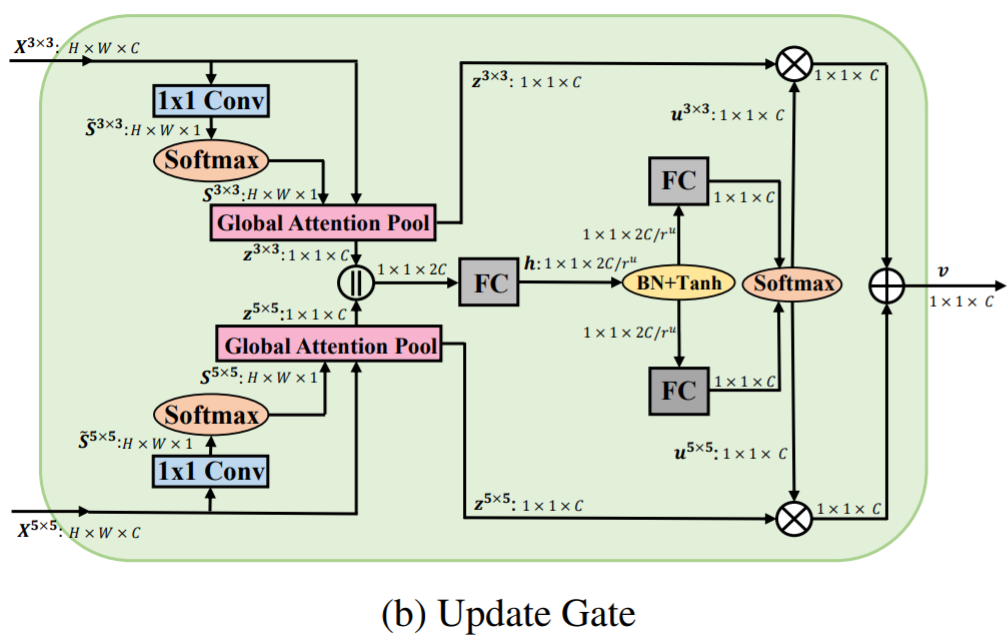spatial注意力，pooling：作者采用全局上下文建模来计算spatial-wise权重对于每个像素位置。对于给定的特征图 X 3 × 3 ∈ R H × W × C \mathbf{X}^{3\times 3}\in \mathbb{R}^{H\times W\times C} ,一个 1 × 1 1\times1 卷积核沿着channel维度进行收缩得到spatial注意力特征图 S ~ 3 × 3 ∈ R H × W × 1 \tilde{\mathbf{S}}^{3\times 3}\in \mathbb{R}^{H\times W\times 1} , 然后一个softmax函数归一化它得到最终的spatial注意力特征图 S 3 × 3 ∈ R H × W × 1 \mathbf{S}^{3\times 3}\in \mathbb{R}^{H\times W\times 1} , 每一个元素为:
S i , j , 1 3 × 3 = e S ~ i , j , 1 3 × 3 ∑ x = 1 H ∑ y = 1 W e S ~ x , y , 1 3 × 3 \mathbf{S}^{3\times 3}_{i,j,1}=\frac{e^{\tilde{\mathbf{S}}^{3\times 3}_{i,j,1}}}{\sum_{x=1}^{H}\sum_{y=1}^{W}e^{\tilde{\mathbf{S}}^{3\times 3}_{x,y,1}}}

z c 3 × 3 = ∑ x = 1 H ∑ y = 1 W X x , y , c 3 × 3 ∗ S x , y , c 3 × 3 \mathbf{z}_{c}^{3\times 3}=\sum_{x=1}^{H}\sum_{y=1}^{W} \mathbf{X}^{3\times 3}_{x,y,c}*\mathbf{S}^{3\times 3}_{x,y,c}

channel注意力： 为了保持信息的完整性，作者连接 z 3 × 3 \mathbf{z}^{3\times 3} z 5 × 5 \mathbf{z}^{5\times 5} 作为输入。 然后它被转换为一个隐藏表示 h ∈ R 1 × 1 × 2 ∗ C / r u \mathbf{h}\in \mathbb{R}^{1\times 1\times {2*C}/{r}^{u}} , 这个 h \mathbf{h} 通常是一个稠密的特征图通过设置一个缩减因子 r u r^{u} 来得到更好的效率. 这可以通过一个全连接层和非线性来实现：
h = tanh ⁡ ( B N ( W [ z 3 × 3 ∥ z 5 × 5 ] ) + b ) \mathbf{h}=\tanh(BN(\mathbf{W}[\mathbf{z}^{3\times 3}\parallel \mathbf{z}^{5\times 5}])+\mathbf{b})

u ~ 3 × 3 = W 3 × 3 h + b 3 × 3 , u ~ 5 × 5 = W 5 × 5 h + b 3 × 3 \tilde{\mathbf{u}}^{3\times 3}=\mathbf{W}^{3 \times 3}\mathbf{h}+\mathbf{b}^{3\times 3}, \tilde{\mathbf{u}}^{5\times 5}=\mathbf{W}^{5 \times 5}\mathbf{h}+\mathbf{b}^{3\times 3}

u 3 × 3 = e u ~ 3 × 3 e u ~ 3 × 3 + e u ~ 5 × 5 , u 5 × 5 = e u ~ 5 × 5 e u ~ 3 × 3 + e u ~ 5 × 5 \mathbf{u}^{3\times 3}=\frac{e^{\tilde{\mathbf{u}}^{3\times 3}}}{e^{\tilde{\mathbf{u}}^{3\times 3}}+e^{\tilde{\mathbf{u}}^{5\times 5}}},\mathbf{u}^{5\times 5}=\frac{e^{\tilde{\mathbf{u}}^{5\times 5}}}{e^{\tilde{\mathbf{u}}^{3\times 3}}+e^{\tilde{\mathbf{u}}^{5\times 5}}}
u 3 × 3 \mathbf{u}^{3\times 3} u 5 × 5 \mathbf{u}^{5\times 5} 可以被看作是聚合多尺度全局上下文特征的比例。对于 z 3 × 3 \mathbf{z}^{3\times 3} z 5 × 5 \mathbf{z}^{5\times 5} 加权聚合 是更新门的最终输出:
v c = u c 3 × 3 ⋅ z c 3 × 3 + u c 5 × 5 ⋅ z c 5 × 5 , u c 3 × 3 + u c 5 × 5 = 1 \mathbf{v}_{c}=\mathbf{u}_{c}^{3\times 3}\cdot \mathbf{z}_{c}^{3\times 3}+\mathbf{u}_{c}^{5\times 5}\cdot \mathbf{z}_{c}^{5\times 5}, \mathbf{u}_{c}^{3\times 3}+\mathbf{u}_{c}^{5\times 5}=1

#### Forget Gate （遗忘门）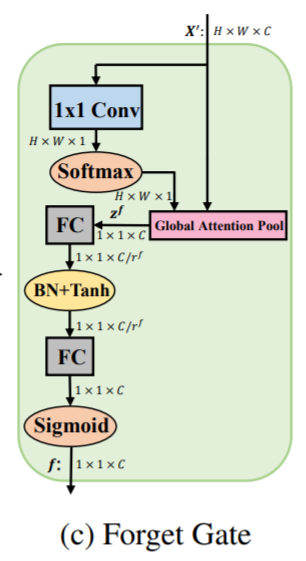spatial注意力和pooling：对于给定的特征图 X ′ ∈ R H × W × C \mathbf{X}^{'}\in \mathbb{R}^{H\times W\times C} , 作者使用与更新门相同的全局注意力池化机制，然后一个channel描述器 z f ∈ R 1 × 1 × C \mathbf{z}^{f}\in \mathbb{R}^{1\times 1\times C} 可以被得到
channel注意力： 为了满足加权衰减每一个通道的需求，每一个通道的权重应该在 ( 0 , 1 ) (0,1) 之间，因此作者采用SE模块，堆叠两个连续的瓶颈形状的全连接层并且使用sigmoid函数作为结尾。不同于SE模块，作者嵌入了批标准化来缓解优化问题并且替换ReLU为 tanh ⁡ \tanh 函数为非线性。总之，对于输入 z f \mathbf{z}^{f} ，一系列的转换如下所示:
f = σ ( W 2 f ( tanh ⁡ ( B N ( W 1 f z f + b 1 f ) ) ) + b 2 f ) \mathbf{f}=\sigma(\mathbf{W}_{2}^{f}(\tanh (BN(\mathbf{W}_{1}^{f}\mathbf{z}^{f}+\mathbf{b}^{f}_{1})))+\mathbf{b}^{f}_{2})

## 2.3 Macro-architecture （宏结构）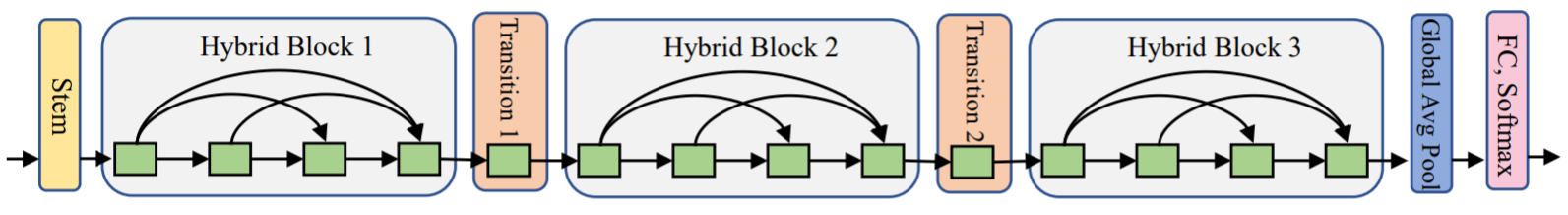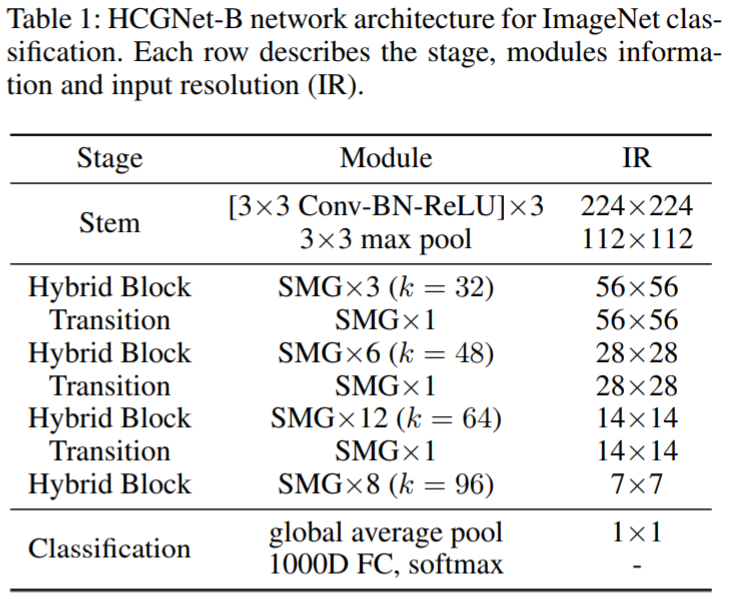hybrid 块和transition layer 都采用SMG模块但是使用不同的超参数设置。我们仅仅堆叠一个SMG模块来建立transition layer，一个压缩因子 θ = 0.5 \theta=0.5 被用来减少channel数量，即 C = θ C ~ C=\theta \tilde{C} 。对于每个SMG模块，作者在hybrid 块中设置 g = 4 g=4 , α = 4 \alpha=4 r u = r f = 2 r^{u}=r^{f}=2 ，同时在transition layers中设置 g = 1 g=1 , α = 1.5 \alpha=1.5 , S = 2 S=2 r u = r f = 4 r^{u}=r^{f}=4 。注意作者应用标准的卷积在transition layers来获得最佳的特征提取能力，应用组卷积在hybrid block中来更好的权衡效率和表现。与hybrid block相比，作者设置更小的乘子 α \alpha 和更大的缩减因子 r u r^{u} r f r^{f} 来提高效率，由于在transition layers的特征图具有更多的channel数量 。

# 实验结果

## CIFAR的实验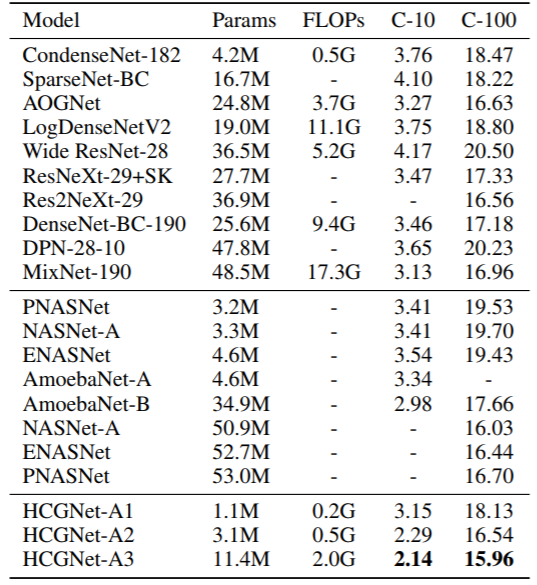### 人工设计的网络对比

DenseNet-190每一个dense块具有31个模块，HCGNet-A2每一个hybrid block仅有8个模块，因此减少了93%的冗余性但是具有显著的准确率增益。此外，HCGNet-A2显著地超过了其他稀疏化的变体，例如LogDenseNet，SparseNet和CondenseNet，表明了作者对于DenseNet的优化比稀疏化方法更加有效。HCGNet-A2使用 16 × 16\times 更少的参数超过了MixNet-190，它代表了ResNet和DenseNet最泛化的形式。HCGNet-A2使用 8 × 8\times 更少的参数但是胜过了AOGNet，它是SOTA人工设计的网络通过分层和组合式的特征聚合。因此，作者提出的嵌套聚合是最佳的方法相比于其他ResNet和DenseNet的结合体和变体。

### 自动搜索的网络对比

HCGNet比自动搜索的网络更加有效率。在小规模的设置下，HCGNet-A2分别获得了 1%3% 的CIFAR-10和CIFAR-100错误率减少相对于其他的搜索获得的网络。此外，HCGNet-A1获得了优越的表现伴随着前所未有的效率。在大规模的设置下，HCGNet-A3完成了最佳的效果伴随着最小的复杂度。有点惊讶的是，HCGNet-A3超过了最有竞争力的NASNet-A仅仅需要其22%的参数量。

## ImageNet的实验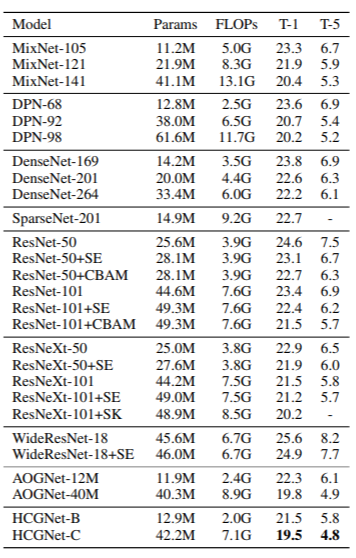### 与流行的网络对比

HCGNets凭借更少或相近的复杂度在流行的网络中获得了最佳的表现，包括Top-1和Top-5错误率。DenseNet-169堆叠了4个dense块，分别有6.12.32.32个模块，HCGNet-B利用更浅层的设计，分别有3,6,12,8个模块对于4个hybrid block，因此减少了88%的冗余性但是获得了2.3%的绝对增益。此外，HCGNets产生了显著更好的结果相对于DenseNet，MixNet以及DPN家族在相似复杂度的条件下。使用显著的 4.6 × 4.6\times 更少的FLOPs，HCGNet-B可以超过SparseNet-201，它是SOTA DenseNet变体。HCGNet家族获得了更好的表现相对于ResNet，ResNeXt以及WideResNet家族以及它们的attention变体，它们代表了实践中广泛使用的模型。卓越地，HCGNets超过了先前的SOTA AOGNet在不同的模型规格下，进一步展示HCGNet设计的优越性。

### 模型可解释性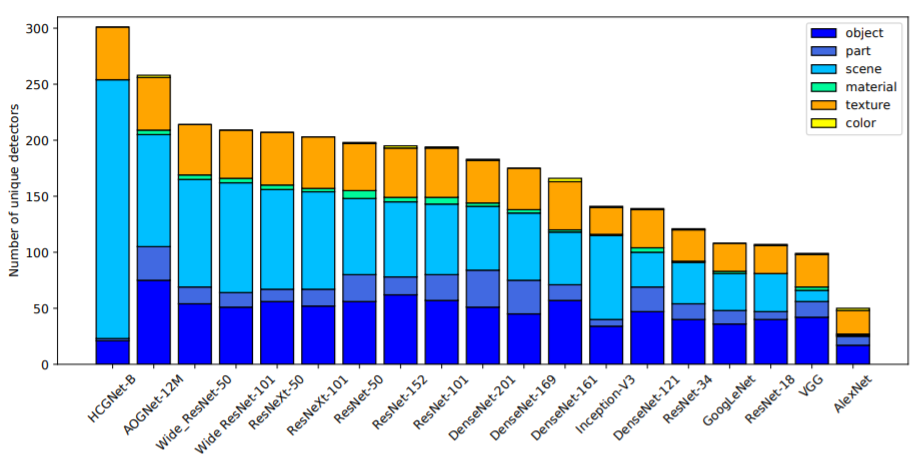### 对抗鲁棒性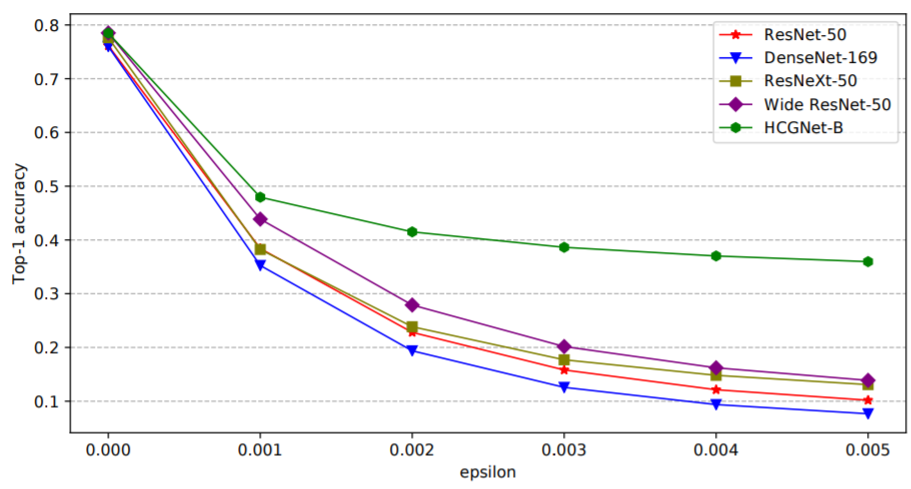### 目标检测和实例分割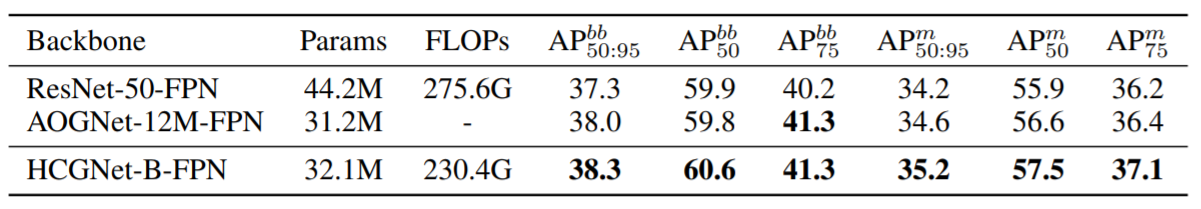03-023474
02-281563
11-191822
08-19228
03-184918
02-122566
02-20608
11-044215
03-28599
03-079653
10-051462
03-113795
08-083万+
02-261748
04-191万+
04-204657
02-211万+
07-03237
02-18532

### “相关推荐”对你有帮助么？

•非常没帮助
•没帮助
•一般
•有帮助
•非常有帮助被折叠的  条评论 为什么被折叠?到【灌水乐园】发言winycg

¥2 ¥4 ¥6 ¥10 ¥20余额支付 (余额：-- )扫码支付获取中扫码支付点击重新获取扫码支付1.余额是钱包充值的虚拟货币，按照1:1的比例进行支付金额的抵扣。
2.余额无法直接购买下载，可以购买VIP、C币套餐、付费专栏及课程。余额充值